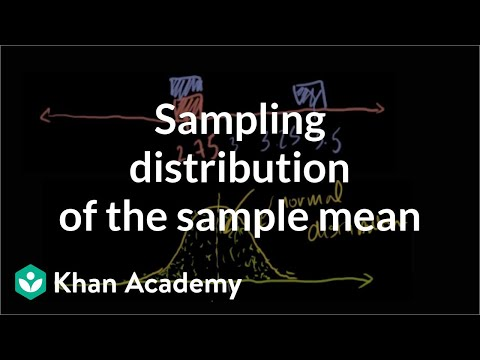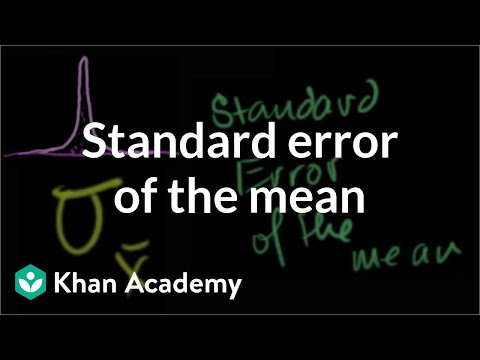# 7.1: The Central Limit Theorem for Sample Means (Averages)

$$\newcommand{\vecs}{\overset { \rightharpoonup} {\mathbf{#1}} }$$ $$\newcommand{\vecd}{\overset{-\!-\!\rightharpoonup}{\vphantom{a}\smash {#1}}}$$$$\newcommand{\id}{\mathrm{id}}$$ $$\newcommand{\Span}{\mathrm{span}}$$ $$\newcommand{\kernel}{\mathrm{null}\,}$$ $$\newcommand{\range}{\mathrm{range}\,}$$ $$\newcommand{\RealPart}{\mathrm{Re}}$$ $$\newcommand{\ImaginaryPart}{\mathrm{Im}}$$ $$\newcommand{\Argument}{\mathrm{Arg}}$$ $$\newcommand{\norm}{\| #1 \|}$$ $$\newcommand{\inner}{\langle #1, #2 \rangle}$$ $$\newcommand{\Span}{\mathrm{span}}$$ $$\newcommand{\id}{\mathrm{id}}$$ $$\newcommand{\Span}{\mathrm{span}}$$ $$\newcommand{\kernel}{\mathrm{null}\,}$$ $$\newcommand{\range}{\mathrm{range}\,}$$ $$\newcommand{\RealPart}{\mathrm{Re}}$$ $$\newcommand{\ImaginaryPart}{\mathrm{Im}}$$ $$\newcommand{\Argument}{\mathrm{Arg}}$$ $$\newcommand{\norm}{\| #1 \|}$$ $$\newcommand{\inner}{\langle #1, #2 \rangle}$$ $$\newcommand{\Span}{\mathrm{span}}$$$$\newcommand{\AA}{\unicode[.8,0]{x212B}}$$

Suppose X is a random variable with a distribution that may be known or unknown (it can be any distribution).
Using a subscript that matches the random variable, suppose:

1. μX = the mean of X
2. σX = the standard deviation of X

If you draw random samples of size n, then as n increases, the random samples$\displaystyle\overline{{X}}$ which consists of sample means, tend to be normally distributed.$\displaystyle\overline{{X}}$ ~ N ($\displaystyle{\mu}_{x}$,$\displaystyle\frac{{\sigma_{x}}}{{\sqrt{n}}}$)

The central limit theorem for sample means says that if you keep drawing larger and larger samples (such as rolling one, two, five, and finally, ten dice) and calculating their means, the sample means form their own normal distribution (the sampling distribution). The normal distribution has the same mean as the original distribution and a variance that equals the original variance divided by, the sample size. The variable n is the number of values that are averaged together, not the number of times the experiment is done.

To put it more formally, if you draw random samples of size n, the distribution of the random variable$\displaystyle\overline{{X}}$, which consists of sample means, is called the sampling distribution of the mean. The sampling distribution of the mean approaches a normal distribution as the sample size n increases.

The random variable$\displaystyle\overline{{X}}$ has a different z-score associated with it from that of the random variable X. The mean$\displaystyle\overline{x}$ is the value of$\displaystyle\overline{X}$ in one sample.

z =$\displaystyle\frac{{\overline{x}-{\mu}_{x}}}{{\frac{{{\sigma}_{x}}}{{\sqrt{n}}}}}$$\displaystyle{\mu}_{x}$ =$\displaystyle{\mu}_{\overline{x}}$  (mean of X = mean of$\displaystyle\overline{X}$. )$\displaystyle{\sigma}_{\overline{x}} = {{\frac{{{\sigma}_{x}}}{{\sqrt{n}}}}}$ = standard deviation of$\displaystyle\overline{{X}}$ and is called the standard error of the mean.

 Guide for TI-Calculator: To find probabilities for means on the TI-calculator, follow these steps: “2nd”“DISTR”“2: normalcdf”$\displaystyle\text{ normalcdf ( lower value, upper value, mean,} \frac{\text{standard deviation}}{\sqrt{\text{sample size}}} \text{)} \$ where: mean is the mean of the original distribution, standard deviation is the standard deviation of the original distribution sample size

### Example 1

An unknown distribution has a mean of 90 and a standard deviation of 15. Samples of size n = 25 are drawn randomly from the population.

1. Find the probability that the sample mean is between 85 and 92.
2. Find the value that is two standard deviations above the expected value, 90, of the sample mean.

### Solution

lower value = 85, upper value = 92, mean${\mu}$ = 90, std dev${\sigma}$ = 15, sample size = 25.

1. In this example, the probability that the sample mean is btw 85 and 92 = area btw 85 and 92.Since 25 samples are drawn,$\displaystyle{\mu}_{x}$ = mean = 90,$\displaystyle{\sigma}_{x}$ =$\displaystyle\frac{{\text{std dev}}}{{\text{sample size}}}$ =$\displaystyle\frac{{15}}{{\sqrt{25}}}$.By using TI-83/84, normalcdf(85, 92, 90,$\displaystyle\frac{{15}}{{\sqrt{25}}}$ ) = 0.0697
2. To find the value that is two standard deviations above the expected value 90, use the formula:
value =$\displaystyle{\mu}_{x}$ + (# of STD DEV)$\displaystyle\left(\frac{{{\sigma}_{x}}}{{\sqrt{n}}}\right)$
Value that is 2 std dev above 90
= 90 + 2 ($\displaystyle\frac{{15}}{{\sqrt{25}}}$ )
= 96
The value that is two standard deviations above the expected value is 96.
(Note: The standard error of the mean is$\displaystyle\frac{{\sigma}}{{\sqrt{n}}}$$\displaystyle\frac{{15}}{{\sqrt{25}}}$ = 3. )

Recall that the standard error of the mean ($\displaystyle\frac{{\sigma}}{{\sqrt{n}}}$) is a description of how far (on average) that the sample mean will be from the population mean in repeated simple random samples of size n.A YouTube element has been excluded from this version of the text. You can view it online here: http://pb.libretexts.org/esm/?p=98

### Try It

An unknown distribution has a mean of 45 and a standard deviation of 8. Samples of size n = 30 are drawn randomly from the population. Find the probability that the sample mean is between 42 and 50.

[practice-area rows=”2″][/practice-area]
TI-Calculator: normalcdf(42, 50, 45,$\displaystyle\frac{{8}}{{\sqrt{30}}}$)
P(42 <$\displaystyle\overline{x}$ < 50) = 0.9797[/hidden-answer]

### Example 2

The length of time, in hours, it takes an “over 40” group of people to play one soccer match is normally distributed with a mean of 2 hours and a standard deviation of 0.5 hours. A sample of size n = 50 is drawn randomly from the population. Find the probability that the sample mean is between 1.8 hours and 2.3 hours.

Solution:
In this example, mean${\mu}$ = 2, std dev${\sigma}$ = 0.5, sample size n = 50

Let$\displaystyle\overline{X}$ = the mean time, in hours, it takes to play one soccer match.

We are looking for P(1.8 <$\displaystyle\overline{x}$ < 2.3).

TI-Calculator:    normalcdf (1.8,2.3, 2,$\displaystyle\frac{0.5}{\sqrt{50}}$)

The probability that the mean time is between 1.8 hours and 2.3 hours
=   P(1.8 <$\displaystyle\overline{x}$ < 2.3)
=   0.9977.

### Try It

The length of time taken on the SAT for a group of students is normally distributed with a mean of 2.5 hours and a standard deviation of 0.25 hours. A sample size of n = 60 is drawn randomly from the population. Find the probability that the sample mean is between two hours and three hours.

[practice-area rows=”1″][/practice-area]
normalcdf( 2, 3, 2.5,$\displaystyle\frac{{0.25}}{{\sqrt{60}}}$
P(2 <$\displaystyle\overline{x}$ < 3) = 1[/hidden-answer]

 Guide for TI-Calculator: To find percentiles for means on the calculator, follow these steps. 2nd DIStR3:invNormk = invNorm (area to the LEFT of k, mean,$\displaystyle{\frac{{\text{standard deviation}}}{{\sqrt{\text{sample size}}}}}$) where: k = the kth percentile, mean is the mean of the original distribution, standard deviation is the standard deviation of the original distribution, sample size = n

### Example 3

In a recent study reported Oct. 29, 2012 on the Flurry Blog, the mean age of tablet users is 34 years. Suppose the standard deviation is 15 years. Take a sample of size n = 100.

1. What are the mean and standard deviation for the sample mean ages of tablet users?
2. What does the distribution look like?
3. Find the probability that the sample mean age is more than 30 years (the reported mean age of tablet users in this particular study).
4. Find the 95th percentile for the sample mean age (to one decimal place).

### Solution

In this example, mean${\mu}$ = 34 years, std dev${\sigma}$ = 15 years, sample size n = 100

1. Since the sample mean tends to target the population mean, the mean for the sample mean ages of tablet users μX = μ = 34.
The sample standard deviation for the sample mean ages is given by$\displaystyle\frac{{\sigma}}{{\sqrt{n}}}=\frac{{15}}{{\sqrt{100}}}=\frac{{15}}{{10}}={1.5}$
2. The central limit theorem states that for large sample sizes(n), the sampling distribution will be approximately normal.
3. TI-Calculator: normalcdf(30,1E99,34,1.5)
The probability that the sample mean age is more than 30 = P(Χ > 30) = 0.9962
4. Let k = the 95th percentile.
TI-Calculator: invNorm(0.95, 34,$\displaystyle\frac{{15}}{{\sqrt{100}}}$)
k = 95th percentile = 36.5.

### Try It

In an article on Flurry Blog, a gaming marketing gap for men between the ages of 30 and 40 is identified. You are researching a startup game targeted at the 35-year-old demographic. Your idea is to develop a strategy game that can be played by men from their late 20s through their late 30s. Based on the article’s data, industry research shows that the average strategy player is 28 years old with a standard deviation of 4.8 years. You take a sample of 100 randomly selected gamers. If your target market is 29- to 35-year-olds, should you continue with your development strategy?
You need to determine the probability for men whose mean age is between 29 and 35 years of age wanting to play a strategy game (also known as P(29 <$\displaystyle\overline{x}$ < 35). )

TI-Calculator: normalcdf = 0.0186 (29, 35, 28,$\displaystyle\frac{{4.8}}{{\sqrt{100}}}$)

P(29 <$\displaystyle\overline{x}$ < 35) = 0.0186

You can conclude there is approximately a 2% chance that your game will be played by men whose mean age is between 29 and 35.[/hidden-answer]

### Example 4

The mean number of minutes for app engagement by a tablet user is 8.2 minutes. Suppose the standard deviation is one minute. Take a sample of 60.

1. What are the mean and standard deviation for the sample mean number of app engagement by a tablet user?
2. What is the standard error of the mean?
3. Find the 90th percentile for the sample mean time for app engagement for a tablet user. Interpret this value in a complete sentence.
4. Find the probability that the sample mean is between eight minutes and 8.5 minutes.

### Solution

In this example, mean${\mu}$ = 8.2, std dev${\sigma}$ = 1, sample size n = 60

1. The mean for the sample mean number of app engagement by a tablet user =$\displaystyle{\mu}_{\overline{x}}={\mu}=8.2$.
2. The std dev for the sample mean number of app engagement by a tablet user =$\displaystyle{\sigma}_{\overline{x}}=\frac{{\sigma}}{{\sqrt{n}}}=\frac{{1}}{{\sqrt{60}}} = 0.13$
***************************************************************************************************************
This allows us to calculate the probability of sample means of a particular distance from the mean, in repeated samples of size 60.
***************************************************************************************************************
3. Let k = the 90th percentile.
TI-Calculator: invNorm(0.9, 8.2,$\displaystyle\frac{{1}}{{\sqrt{60}}}$)
k = the 90th percentile = 8.37.
90 percent of the average app engagement time for table users is less than 8.37 minutes.
4. TI-Calculator: normalcdf(8, 8.5, 8.2$\displaystyle\frac{{1}}{{\sqrt{60}}}$)
P(8 <$\displaystyle\overline{x}$ < 8.5) = 0.9293A YouTube element has been excluded from this version of the text. You can view it online here: http://pb.libretexts.org/esm/?p=98

### Try It

Cans of a cola beverage claim to contain 16 ounces. The amounts in a sample are measured and the statistics are n = 34,$\displaystyle\overline{x}$ = 16.01 ounces. If the cans are filled so that μ = 16.00 ounces (as labeled) and σ= 0.143 ounces, find the probability that a sample of 34 cans will have an average amount greater than 16.01 ounces. Do the results suggest that cans are filled with an amount greater than 16 ounces?

Ti-Calculator: normalcdf(16.01, 1E99, 16,$\displaystyle\frac{{0.143}}{{\sqrt{34}}}$ )

P($\displaystyle\overline{x}$ > 16.01) = 0.3417

Since there is a 34.17% probability that the average sample weight is greater than 16.01 ounces, we should be skeptical of the company’s claimed volume. If I am a consumer, I should be glad that I am probably receiving free cola. If I am the manufacturer, I need to determine if my bottling processes are outside of acceptable limits.[/hidden-answer]

## References

Baran, Daya. “20 Percent of Americans Have Never Used Email.”WebGuild, 2010. Available online at http://www.webguild.org/20080519/20-...ver-used-email (accessed May 17, 2013).

Data from The Flurry Blog, 2013. Available online at http://blog.flurry.com (accessed May 17, 2013).

Data from the United States Department of Agriculture.